Plotting¶

Scatter Graphs¶

Simple Plot¶

After executing a census one can use the plot function to create a scatter graph of results. The x axis is the location along the genome (with ordered chromosomes or contigs appearing sequentially) and the y axis is the value of the censused region according to the strategy used. The example below plots GC content ratio across the first three chromosomes of the hs37d5 reference sequence, with a window size of 100,000 and a step or overlap of 50,000. Note that the plot title may be specified with the title keyword argument.

from goldilocks import Goldilocks
from goldilocks.strategies import GCRatioStrategy

sequence_data = {
"my_sequence": {"file": "/store/ref/hs37d5.1-3.fa.fai"},
}
g = Goldilocks(GCRatioStrategy(), sequence_data, length="100K", stride="50K", is_faidx=True)
g.plot(title="GC Content over hs37d5 Chr1-3")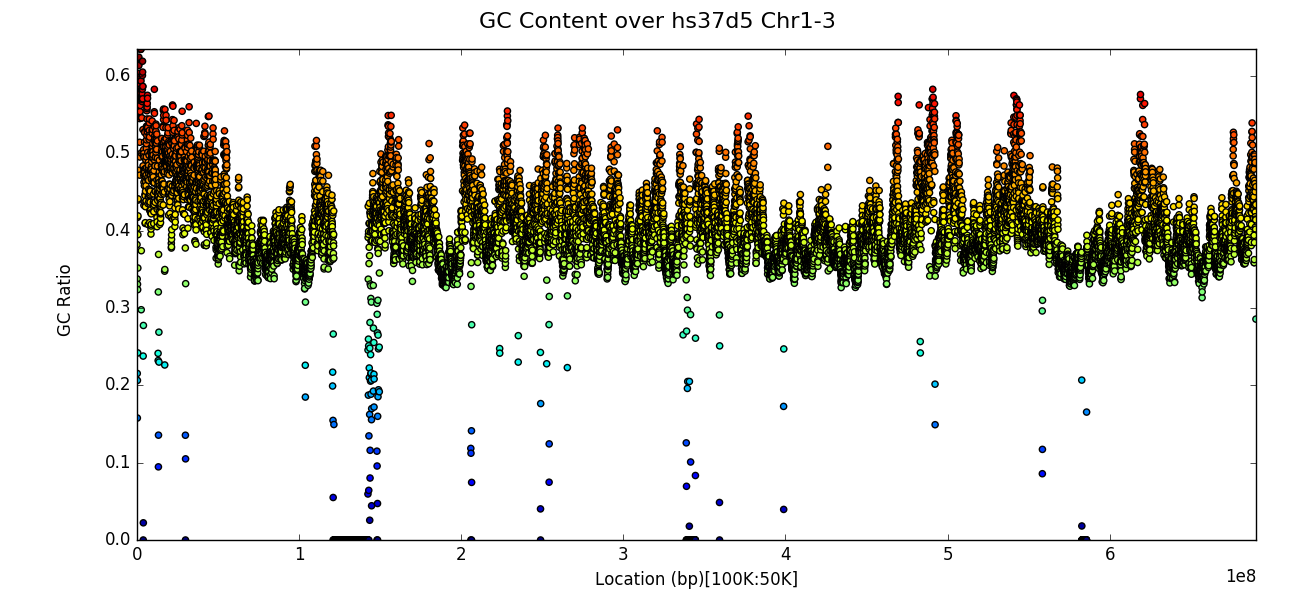Line Graphs¶

Plot multiple contigs or chromosomes from one sample¶

For long genomes or a census with a small window size, simple plots as shown in the previous section can appear too crowded and thus difficult to extract information from. One can instead plot, for a given input sample, a panel of census region data, by chromosome by specifying the name of the sample as the first parameter to the plot function as per the example below:

from goldilocks import Goldilocks
from goldilocks.strategies import GCRatioStrategy

sequence_data = {
"hs37d5": {"file": "/store/ref/hs37d5.1-3.fa.fai"},
"GRCh38": {"file": "/store/ref/Homo_sapiens.GRCh38.dna.chromosome.1-3.fa.fai"},
}
g = Goldilocks(GCRatioStrategy(), sequence_data, length="1M", stride="250K", is_faidx=True)
g.plot("hs37d5", title="GC Content over hs37d5 Chr1-3")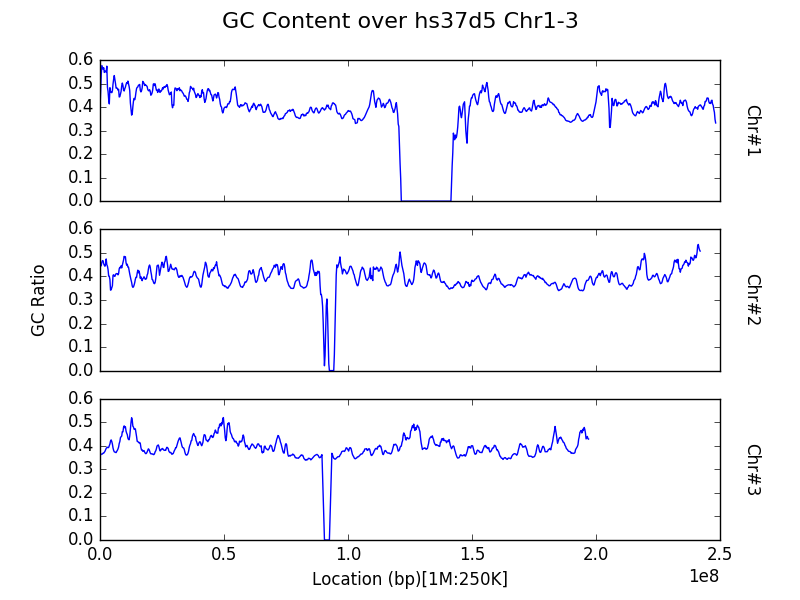Note that both the x and y axes are shared between all panels to avoid the automatic creation of graphics with the potential to mislead readers on a first glance by not featuring the same axes ticks.

Plot a contig or chromosome from multiple samples¶

By default, data within the census is aggregated by region across all input samples (in the sequence_data dictionary) for the entire genome. However, one may be interested in comparisons across samples, rather than between chromosomes in a single sample. One can plot the census results for a specific contig or chromosome for each of the input samples, by specifying the chrom keyword argument to the plot function. Take note that the argument refers to the sequence that appears as the i’th contig of each of the input FASTA and not the actual name or identifier of the chromosome itself.

from goldilocks import Goldilocks
from goldilocks.strategies import GCRatioStrategy

sequence_data = {
"hs37d5": {"file": "/store/ref/hs37d5.1.fa.fai"},
"GRCh38": {"file": "/store/ref/Homo_sapiens.GRCh38.dna.chromosome.1.fa.fai"},
}
g = Goldilocks(GCRatioStrategy(), sequence_data, length="1M", stride="250K", is_faidx=True)
g.plot(chrom=1, title="GC Content over Chr1")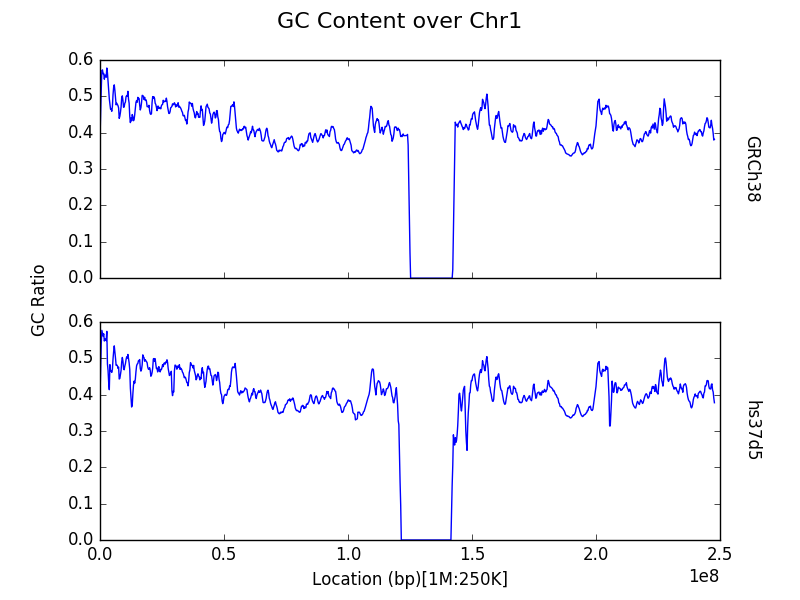Histograms¶

Simple profile (binning) plot¶

Rather than inspection of individual data points, one may want to know how census data behaves as a whole. The plot function provides functionality to profile the results of a census through a histogram. Users can do this by providing a list of bins to the bins keyword argument of the plot function, following a census.

The example below shows the distribution of GC content ratio across the hs37d5 reference sequence for all 100Kbp regions (and step of 50Kbp). The x axis is the bin and the y axis represents the number of censused regions that fell into a particular bin.

from goldilocks import Goldilocks
from goldilocks.strategies import GCRatioStrategy

sequence_data = {
"my_sequence": {"file": "/store/ref/hs37d5.fa.fai"}
}

g = Goldilocks(GCRatioStrategy(), sequence_data,
length="100K", stride="50K", is_faidx=True)

g.plot(bins=[0.0, 0.1, 0.2, 0.3, 0.4, 0.5, 0.6, 0.7, 0.8, 0.9, 1.0],
title="GC Content Profile of hs37d5"
)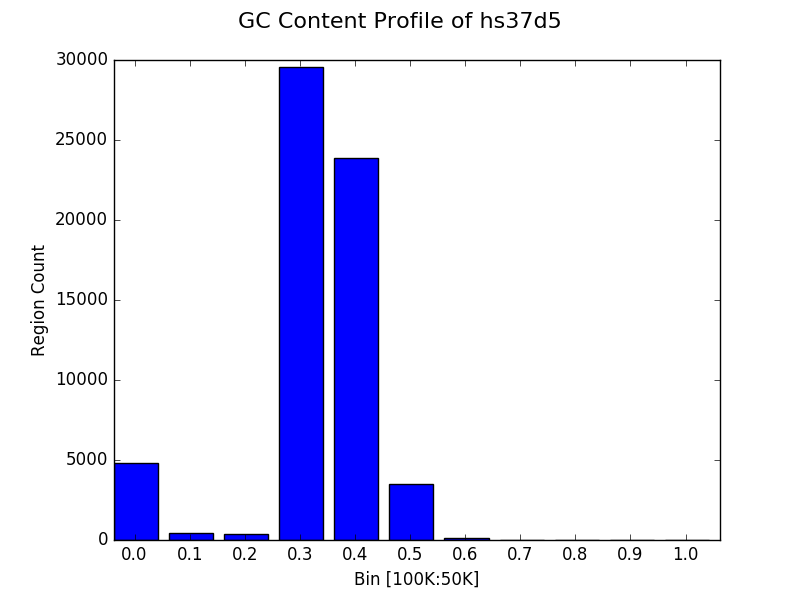Simpler profile (binning) plot¶

It’s trivial to select some sensible bins for the plotting of GC content as we know that the value for each region must fall between 0 and 1. However, many strategies will have an unknown minimum and maximum value and it can thus be difficult to select a suitable binning strategy without resorting to trial and error.

Thus the plot function permits a single integer to be provided to the bins keyword instead of a list. This will automatically create $$N+1$$ equally sized bins (reserving a special bin for 0.0) between 0 and the maximum observed value for the census. It is also possible to manually set the size of the largest bin with the bin_max keyword argument. The following example creates the same graph as the previous subsection, but without explicitly providing a list of bins.

from goldilocks import Goldilocks
from goldilocks.strategies import GCRatioStrategy

sequence_data = {
"my_sequence": {"file": "/store/ref/hs37d5.fa.fai"},
}
g = Goldilocks(GCRatioStrategy(), sequence_data, length="100K", stride="50K", is_faidx=True)
g.plot(bins=10, bin_max=1.0, title="GC Content Profile of hs37d5")

Proportional bin plot¶

Often it can be useful to compare the size of bins in terms of their proportion rather than raw counts alone. This can be accomplished by specifying prop=True to plot. The y axis is now the percentage of all regions that were placed in a particular bin instead of the raw count.

from goldilocks import Goldilocks
from goldilocks.strategies import GCRatioStrategy

sequence_data = {
"my_sequence": {"file": "/store/ref/hs37d5.fa.fai"}
}

g = Goldilocks(GCRatioStrategy(), sequence_data,
length="100K", stride="50K", is_faidx=True)
g.plot(bins=10, bin_max=1.0, prop=True, title="GC Content Profile of hs37d5")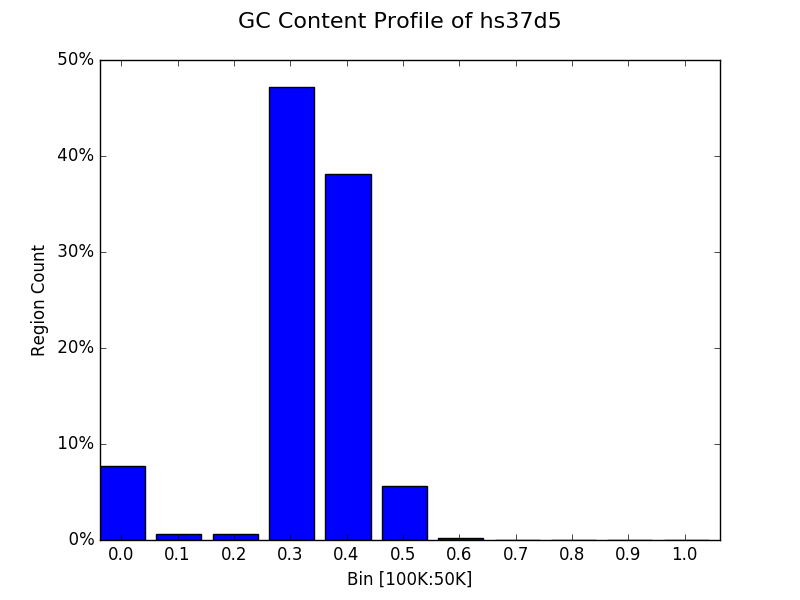Bin multiple contigs or chromosomes from one sample¶

As demonstrated with the line plots earlier, one may also specify a sample name as the first parameter to plot to create a figure with each contig or chromosome’s histogram on an individual panel.

from goldilocks import Goldilocks
from goldilocks.strategies import GCRatioStrategy

sequence_data = {
"my_sequence": {"file": "/store/ref/hs37d5.1-3.fa.fai"}
}

g = Goldilocks(GCRatioStrategy(), sequence_data,
length="100K", stride="50K", is_faidx=True)

g.plot("my_sequence",
bins=10, bin_max=1.0, prop=True, title="GC Content Profiles of hs37d5 Chrs 1-3")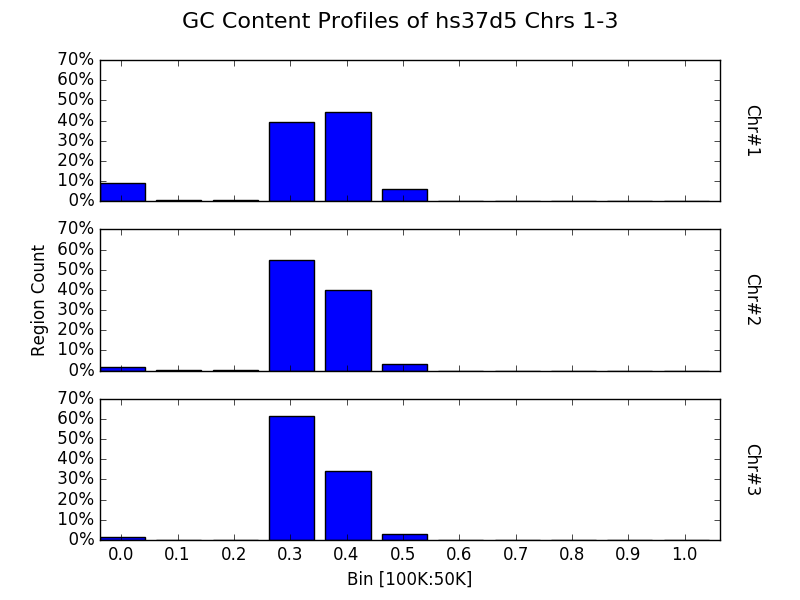Bin a contig or chromosome from multiple samples¶

Similarly, one may want to profile a single contig or chromosome between each input group as previously demonstrated by the line graphs.

from goldilocks import Goldilocks
from goldilocks.strategies import GCRatioStrategy

sequence_data = {
"hs37d5": {"file": "/store/ref/hs37d5.1.fa.fai"},
"GRCh38": {"file": "/store/ref/Homo_sapiens.GRCh38.dna.chromosome.1.fa.fai"}
}

g = Goldilocks(GCRatioStrategy(), sequence_data,
length="100K", stride="50K", is_faidx=True)
g.plot(chrom=1, bins=10, bin_max=1.0, prop=True, title="GC Content Profiles over Chr 1")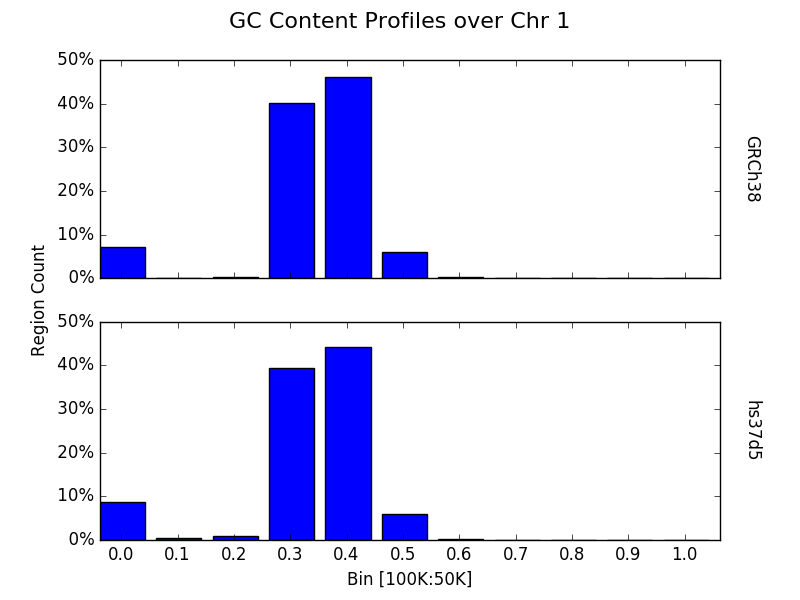Plot data from multiple counting tracks from one sample’s chromosomes¶

The examples thus far have demonstrated plotting the results of a strategy responsible for counting one interesting property. But strategies are capable of counting multiple targets of interest simultaneously. Of course, one may wish to plot the results of all tracks rather than just the totals - especially for cases such as nucleotide counting where the sum of all counts will typically equal the size of the census region! The plot function accepts a list of track names to plot via the tracks keyword argument. Each counting track is then drawn on the same panel for the appropriate chromosome. A suitable legend is automatically placed at the top of the figure.

from goldilocks import Goldilocks
from goldilocks.strategies import NucleotideCounterStrategy

sequence_data = {
"hs37d5": {"file": "/store/ref/hs37d5.1-3.fa.fai"},
}

g = Goldilocks(NucleotideCounterStrategy(["A", "C", "G", "T", "N"]), sequence_data,
length="1M", stride="500K", is_faidx=True, processes=4)
g.plot(group="hs37d5", prop=True, tracks=["A", "C", "G", "T", "N"])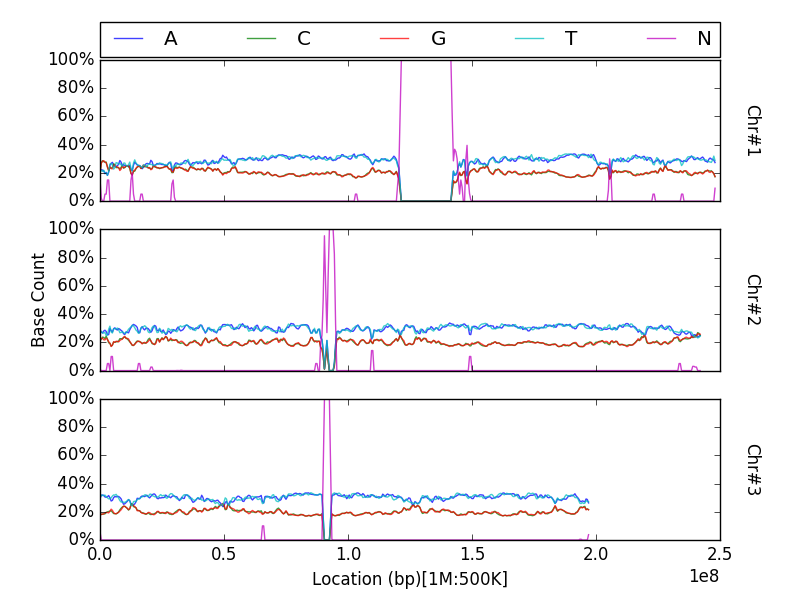Note that prop is not a required argument, but can still be used with the tracks list to plot counts proportionally.

Plot data from multiple counting tracks for one chromosome across many samples¶

As previously demonstrated, one can use the chrom keyword argument for plot to create a figure featuring a panel per input sample, displaying census results for a particular chromosome. Similarly, this feature is supported when plotting multiple tracks with the tracks keyword.

from goldilocks import Goldilocks
from goldilocks.strategies import NucleotideCounterStrategy

sequence_data = {
"hs37d5": {"file": "/store/ref/hs37d5.1.fa.fai"},
"GRCh38": {"file": "/store/ref/Homo_sapiens.GRCh38.dna.chromosome.1.fa.fai"},
}

g = Goldilocks(NucleotideCounterStrategy(["A", "C", "G", "T", "N"]), sequence_data,
length="1M", stride="500K", is_faidx=True, processes=4)
g.plot(chrom=1, prop=True, tracks=["A", "C", "G", "T", "N"])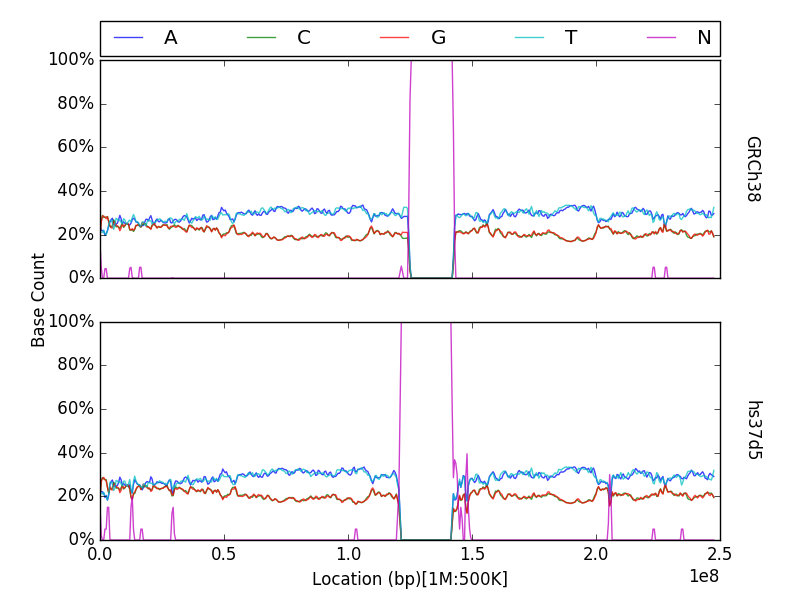Integration with external plotting tools¶

ggplot2¶

Plotting packages such as ggplot2 favour melted” input. The figure below was created using data from Goldilocks as part of our quality control study, the scatter plot compares the density of SNPs between the GWAS and SNP chip studies across the human genome.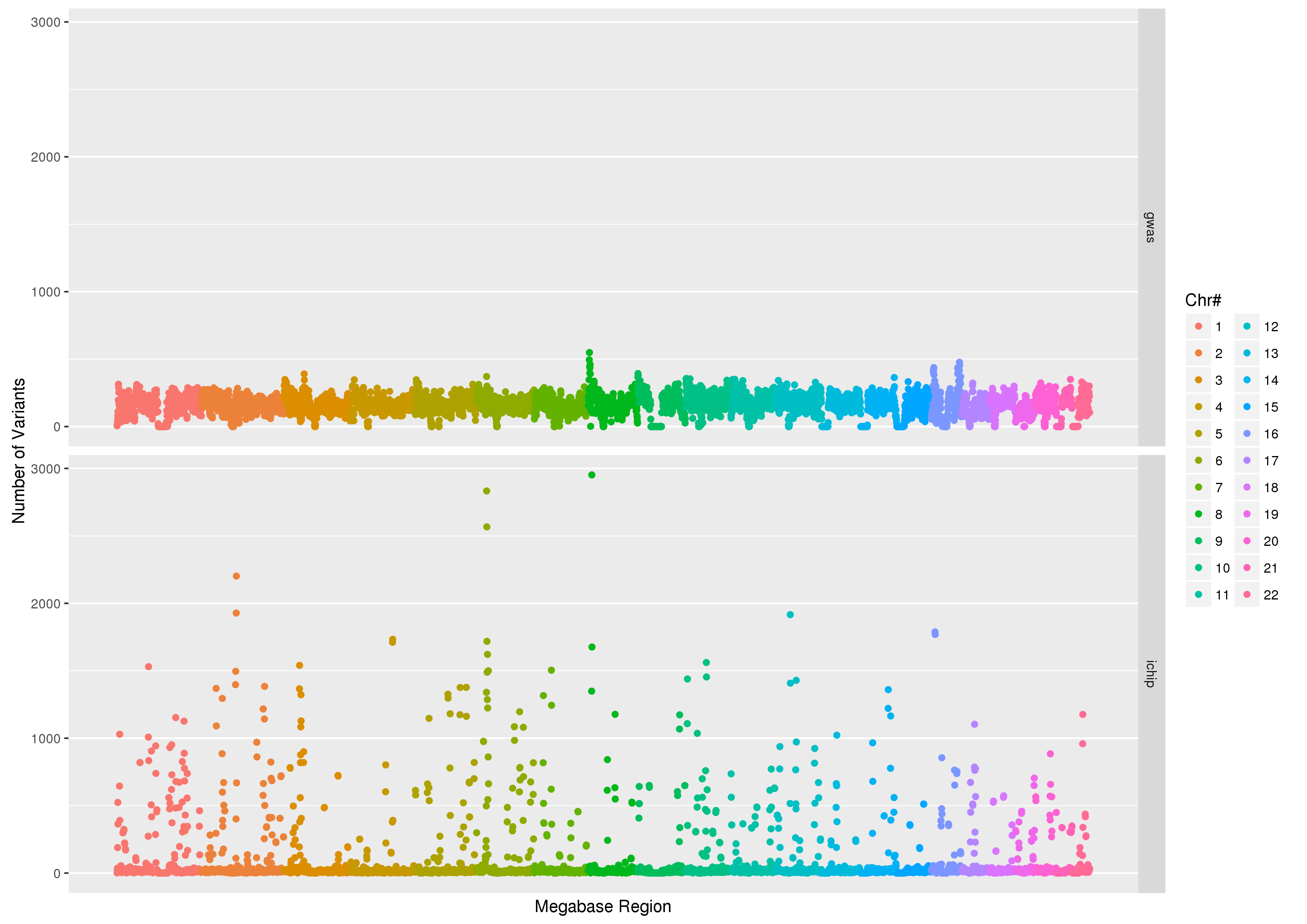Circos¶

Goldilocks has an output format specifically designed to output information for use with the popular and pretty” circos visualisation tool. Below is an example of a figure that can be generated from data gathered by Goldilocks. The figure visualises the selection of regions from our original quality control study. The Python script used to generate the data and the Circos configuration follow.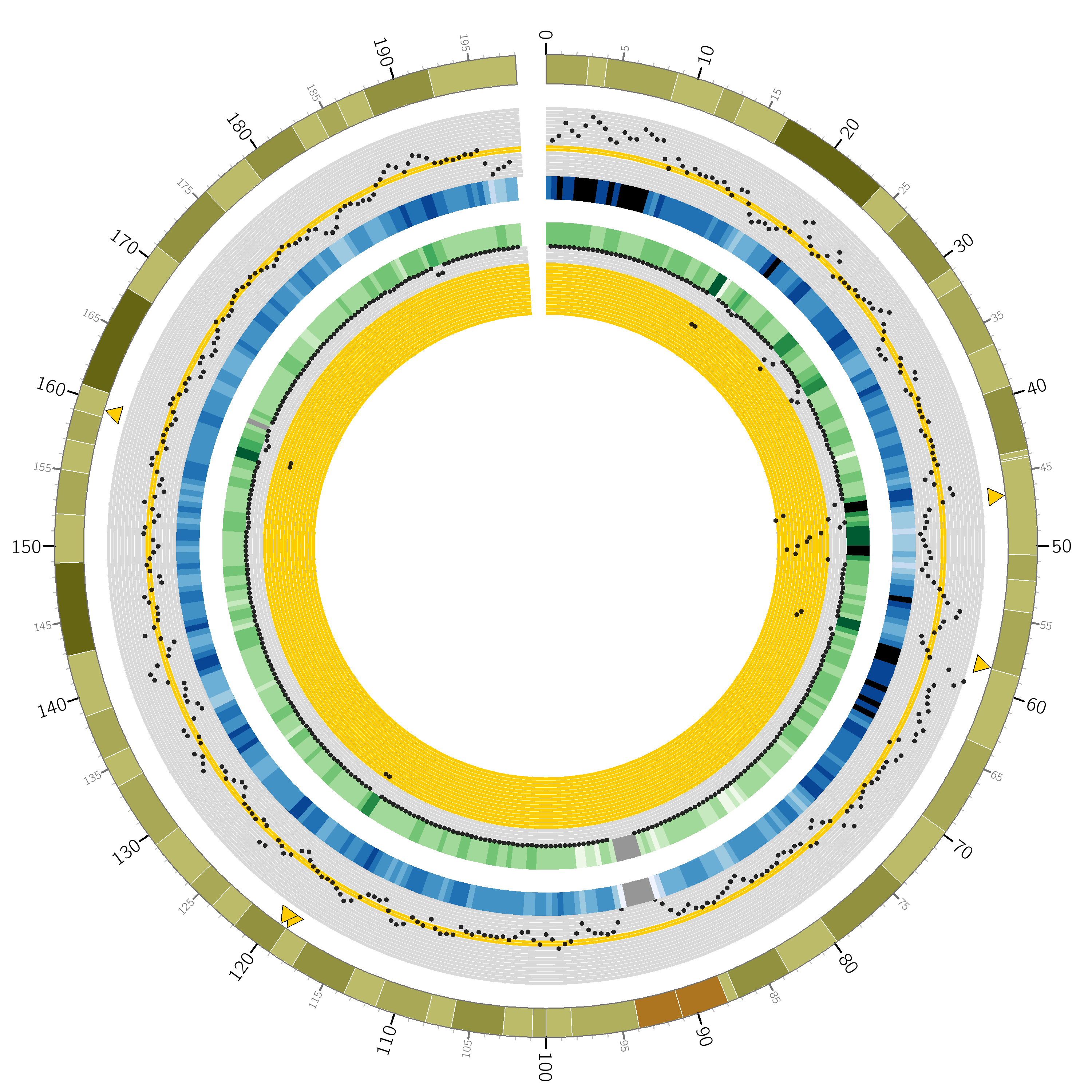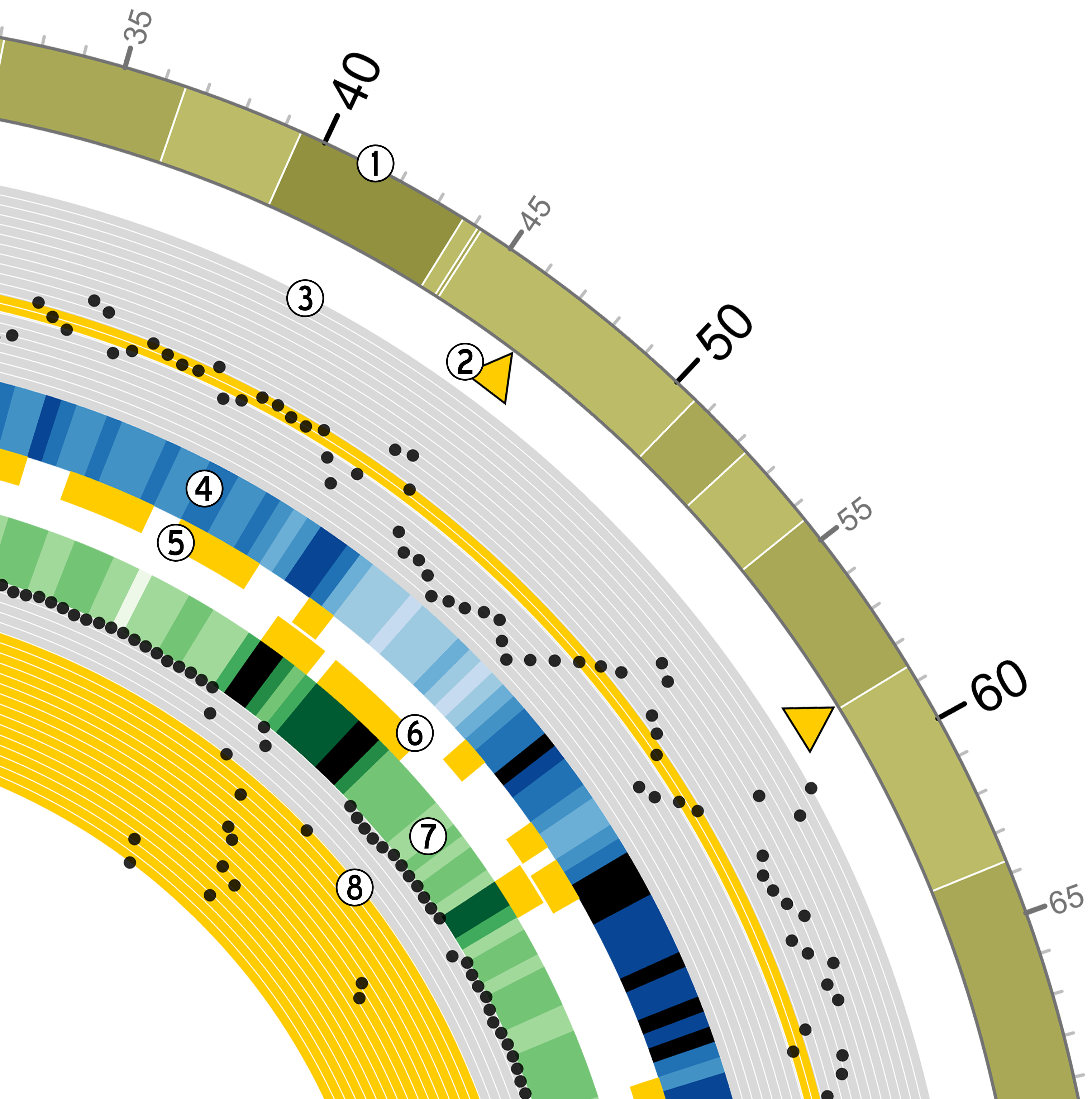Python script

from goldilocks import Goldilocks
from goldilocks.strategies import PositionCounterStrategy

sequence_data = {
"gwas": {"file": "/encrypt/ngsqc/vcf/cd-seq.vcf.q"},
"ichip": {"file": "/encrypt/ngsqc/vcf/cd-ichip.vcf.q"},
}

g = Goldilocks(PositionCounterStrategy(), sequence_data,
length="1M", stride="500K", is_pos_file=True)

# Query for regions that meet all criteria across both sample groups
# The output file goldilocks.circ is used to plot the yellow triangular indicators
g.query("median", percentile_distance=20, group="gwas", exclusions={"chr": })
g.query("max", percentile_distance=5, group="ichip")
g.export_meta(fmt="circos", group="total", value_bool=True, chr_prefix="hs", to="goldilocks.circ")

# Reset the regions selected and saved by queries
g.reset_candidates()

# Export all region counts for both groups individually
# The -all.circ files are used to plot the scatter plots and heatmaps
g.export_meta(fmt="circos", group="gwas", chr_prefix="hs", to="gwas-all.circ")
g.export_meta(fmt="circos", group="ichip", chr_prefix="hs", to="ichip-all.circ")

# Export region counts for the groups where the criteria are met
# The -candidates.circ files are used to plot the yellow 'bricks' that
#   appear between the two middle heatmaps
g.query("median", percentile_distance=20, group="gwas")
g.export_meta(fmt="circos", group="gwas", to="gwas-candidates.circ")
g.reset_candidates()
g.query("max", percentile_distance=5, group="ichip")
g.export_meta(fmt="circos", group="ichip", to="ichip-candidates.circ")
g.reset_candidates()

Circos configuration

# circos.conf
<colors>
gold = 255, 204, 0
</colors>

karyotype = data/karyotype/karyotype.human.hg19.txt
chromosomes_units           = 1000000
chromosomes_display_default = no
chromosomes = hs3;

<ideogram>

<spacing>
default = 0.01r
break   = 2u
</spacing>

# Ideogram position, fill and outline
thickness = 80p
fill      = yes
stroke_color     = dgrey
stroke_thickness = 3p

# Bands
show_bands = yes
band_transparency = 4
fill_bands            = yes
band_stroke_thickness = 2
band_stroke_color     = white

# Labels
show_label     = no
label_font       = default
label_radius     = 1r + 75p
label_size     = 72
label_parallel   = yes
label_case     = upper

</ideogram>

# Ticks
show_ticks          = yes
show_tick_labels    = yes

<ticks>

label_font       = default
label_offset         = 5p
orientation          = out
label_multiplier     = 1e-6
color                = black
chromosomes_display_default = yes

<tick>
spacing = 1u
size = 10p
thickness = 3p
color = lgrey
show_label = no
</tick>

<tick>
spacing = 5u
size = 20p
thickness = 5p
color = dgrey
show_label = yes
label_size = 24p
label_offset = 0p
format = %d
</tick>

<tick>
spacing = 10u
size = 30p
thickness = 5p
color = black
show_label = yes
label_size = 40p
label_offset = 5p
format = %d
</tick>

</ticks>

track_width = 0.05
track_start = 0.95

<plots>
<plot>
type            = scatter
file        = goldilocks.circ
r1      = 0.98r
r0      = 0.95r
orientation = out

glyph = triangle
#glyph_rotation = 180
glyph_size = 50p

color     = gold
stroke_thickness = 2p
stroke_color = black

min = 0
max = 1

</plot>
<plot>
type  = scatter

file    = gwas-all.circ
r1      = 0.95r
r0      = 0.80r

fill = no
fill_color       = black
color = black_a1
stroke_color     = black
glyph            = circle
glyph_size       = 12

<backgrounds>
<background>
color = vlgrey
y0    = 207
</background>
<background>
color = vlgrey
y1    = 207
y0    = 179
</background>
<background>
color = gold
y1    = 179
y0    = 148
</background>
<background>
color = vlgrey
y1    = 145
y0    = 122
</background>
<background>
color = vlgrey
y1    = 122
y0    = 0
</background>
</backgrounds>

<axes>
<axis>
color     = white
thickness = 1
spacing   = 0.05r
</axis>
</axes>
<rules>
<rule>
condition  = var(value) < 1
show = no
</rule>
</rules>
</plot>
<plot>
type    = heatmap
file    = gwas-all.circ

# color list
color   = grey,vvlblue,vlblue,lblue,blue,dblue,vdblue,vvdblue,black
r1      = 0.80r
r0      = 0.75r

scale_log_base = 0.75
color_mapping = 2
min = 1
max = 267 # 95%
</plot>

<plot>
type            = tile
layers_overflow = collapse
file        = gwas-candidates.circ
r1      = 0.7495r
r0      = 0.73r
orientation = in

layers      = 1
margin      = 0.0u
thickness   = 30p

color     = gold
stroke_thickness = 0
stroke_color = gold
</plot>
<plot>
type            = tile
layers_overflow = collapse
file        = ichip-candidates.circ
r1      = 0.73r
r0      = 0.70r
orientation = out

layers      = 1
margin      = 0.0u
thickness   = 30p

color     = gold
stroke_color = gold
</plot>

<plot>
type    = heatmap
file    = ichip-all.circ

# color list
color   = grey,vvlgreen,vlgreen,lgreen,green,dgreen,vdgreen,vvdgreen,black
r1      = 0.70r
r0      = 0.65r

min = 1
max = 1097.71 # 99%
color_mapping = 2
scale_log_base = 0.2

</plot>
<plot>
type  = scatter

file    = ichip-all.circ
r1      = 0.65r
r0      = 0.50r
orientation = in

fill_color       = black
stroke_color     = black
glyph            = circle
glyph_size       = 12
color = black_a1

<backgrounds>
<background>
color = gold
y0    = 379
</background>
<background>
color = vlgrey
y1    = 379
y0    = 49
</background>
<background>
color = vlgrey
y1    = 49
y0    = 0
</background>
</backgrounds>

<axes>
<axis>
color     = white
thickness = 1
spacing   = 0.05r
</axis>
</axes>
<rules>
<rule>
condition  = var(value) < 1
show = no
</rule>
</rules>
</plot>

</plots>

################################################################
# The remaining content is standard and required. It is imported
# from default files in the Circos distribution.
#
# These should be present in every Circos configuration file and
# overridden as required. To see the content of these files,
# look in etc/ in the Circos distribution.

<image>
# Included from Circos distribution.
<<include etc/image.conf>>
</image>

# RGB/HSV color definitions, color lists, location of fonts, fill patterns.
# Included from Circos distribution.
<<include etc/colors_fonts_patterns.conf>>

# Debugging, I/O an dother system parameters
# Included from Circos distribution.
<<include etc/housekeeping.conf>>
anti_aliasing* = no